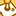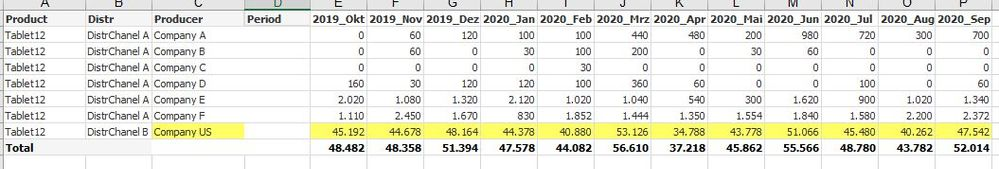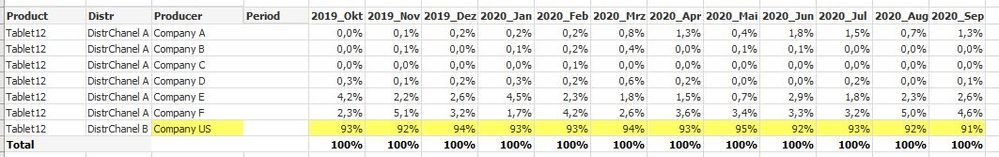# QlikView App Dev

Discussion Board for collaboration related to QlikView App Development.

Announcements
QlikWorld online is next week! REGISTER NOW
cancel
Showing results for
Did you mean:Contributor III

## How to calculate Shares for a dimension AND Time Period

Hello experts,

I have a question of how to calculate Shares % of a Pivot table.

I have follwoing Pivot:The formula here is:  Sum({<Distr = >} [Daten])

But how would I have to change the formula in order to achieve following table:As soon as I use set analysis with "Total" QV calculates  the whole period as total and not the total for each month.

Many thanks for any hint!

Best

Frank

Labels (1)
• ### Shares for Dimension and Time

2 Solutions

Accepted SolutionsSpecialist II

Hi,

You put fields to limit the extent of the TOTAL in angle braces after total - so suggest something like Sum({<Distr = >} [Daten])/Sum({<Distr = >}TOTAL <Period> [Daten]).

Cheers,

Chris.Contributor III
Author

Hi Chris,
Many thanks! That's it.

Sum({<Distr = >} [Daten])/Sum({<Distr = >}TOTAL <YYYY_MM, Quarter, Year> [Daten])

(I have to state <YYYY_MM, Quarter, Year> because I use a 'cyclic group' named "Period", where I switch from "YYYY_MM" to "Quarter" to "Year").

Best
Frank

2 RepliesSpecialist II

Hi,

You put fields to limit the extent of the TOTAL in angle braces after total - so suggest something like Sum({<Distr = >} [Daten])/Sum({<Distr = >}TOTAL <Period> [Daten]).

Cheers,

Chris.Contributor III
Author

Hi Chris,
Many thanks! That's it.

Sum({<Distr = >} [Daten])/Sum({<Distr = >}TOTAL <YYYY_MM, Quarter, Year> [Daten])

(I have to state <YYYY_MM, Quarter, Year> because I use a 'cyclic group' named "Period", where I switch from "YYYY_MM" to "Quarter" to "Year").